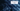# Rotate Image - Leet Code Solution

September 04, 2020## Problem Statement

You are given an n x n 2D matrix representing an image, rotate the image by 90 degrees (clockwise).

You have to rotate the image in-place, which means you have to modify the input 2D matrix directly. DO NOT allocate another 2D matrix and do the rotation.

Example

``````#Input
1 2 3
4 5 6
7 8 9

#Output
7 4 1
8 5 2
9 6 3``````## Solution

Lets visualize a 2x2 matrix above. And, look at the indexes from original position to the target position.

We can easily traverse over the 2D matrix or the image layer by layer. See image below:If you see the highlighted non-yellow color part. Its the outer layer which we will target in first iteration. And, Similarly the next inner layer. So, if we go layer by layer and swapping required indexes. We will get our desired rotated matrix.

Lets look at the index numbers we need to rotate. Since 2D matrix has 4 different sides. Lets look at the indexes we need to swap.``````# First iteration moves
(0,0) to (0,2)
(0,1) to (1,2)
(0,2) to (2,2)
(1,2) to (2,1)
(2,2) to (2,0)
(2,1) to (1,0)
(2,0) to (0,0)
(1,0) to (0,1)``````

Lets look at the code now.

### Code

``````public void rotate(int[][] matrix) {
int n = matrix.length;

for (int layer = 0; layer < n/2; layer ++) {
for (int i=layer; i<n-layer-1; i++) {
//take back before swap
int temp = matrix[layer][i];

matrix[layer][i] = matrix[n-i-1][layer];
matrix[n-i-1][layer] = matrix[n-1-layer][n-i-1];
matrix[n-1-layer][n-i-1] = matrix[i][n-1-layer];
matrix[i][n-1-layer] = temp;
}
}
}``````

Code is pretty small. Its just about playing with indexes.
Notice the first loop. If your 2D matrix is `5x5`, you just need to go for layers `5/2 = 2 layers`.

Go through the code, debug the code and put some print statements for indexes. You will understand it better.

### Complexity

Its simply `O(mxm)`

### Leetcode Submission Result

Runtime: 0 ms, faster than 100.00% of Java online submissions for Rotate Image. Memory Usage: 39.7 MB, less than 46.89% of Java online submissions for Rotate Image.